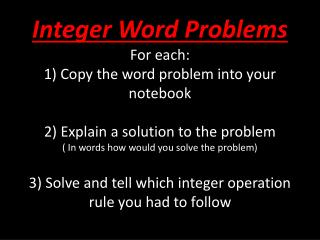DownloadDownload PresentationProblem: Alicia owes \$6 to each of 4 friends. How much money does she owe ? Solution:

# Problem: Alicia owes \$6 to each of 4 friends. How much money does she owe ? Solution:

Download Presentation## Problem: Alicia owes \$6 to each of 4 friends. How much money does she owe ? Solution:

- - - - - - - - - - - - - - - - - - - - - - - - - - - E N D - - - - - - - - - - - - - - - - - - - - - - - - - - -
##### Presentation Transcript

1. Integer Word ProblemsFor each:1) Copy the word problem into your notebook2) Explain a solution to the problem( In words how would you solve the problem)3) Solve and tell which integer operation rule you had to follow

2. Problem: The temperature in Anchorage, Alaska was 8°F in the morning and dropped to -5°F in the evening. What is the difference between these temperatures?* Solution:* Answer/Rule Used:

3. Problem: George owes his friend Jeanne \$3. If he borrows another \$6, how much will he owe her altogether? • Solution: • Answer/Rule Used:

4. Problem: Mrs. Jenson owes \$8,000 on her car loan. Each of her 4 children is willing to pay an equal share of this loan. Using integers, determine how much money each of her children will pay. • Solution: • Answer/Rule Used:

5. Problem: The highest elevation in North America is Mt. McKinley, which is 20,320 feet above sea level. The lowest elevation is Death Valley, which is 282 feet below sea level. What is the difference between these two elevations? • Solution: • Answer/Rule Used

6. Problem: Alicia owes \$6 to each of 4 friends. How much money does she owe? • Solution: • Answer/Rule Used

7. Now Check your answers!!! • If you got it right GREAT!!! • If you got it wrong compare your work, to a person sitting at your table to see where you went wrong in the problem, and learn how to correct your mistakes!!!!

8. Answers: • 1) +8 to 0 is 8, and the distance from 0 to -5 is 5, for a total of 13. • 2) This problem is quite simple: just add \$3 and \$6 and the result is \$9 • 3) -8,000 ÷+4 = -2,000. Each of Mrs. Jenson's four children will pay \$2,000. Let's look at some more examples of dividing integers using the above rules • 4) The opposite of -282 is +282, so we get: +20,320 - -282 = +20,320 + +282 = +20,602 • 5) Owing \$6 can be represented by -6. Thus the problem becomes:   (-6) (+4)